# JP3680746B2 - Control device for infinitely variable continuously variable transmission - Google Patents

## Info

Publication number
JP3680746B2
JP3680746B2 JP2001066289A JP2001066289A JP3680746B2 JP 3680746 B2 JP3680746 B2 JP 3680746B2 JP 2001066289 A JP2001066289 A JP 2001066289A JP 2001066289 A JP2001066289 A JP 2001066289A JP 3680746 B2 JP3680746 B2 JP 3680746B2
Authority
JP
Japan
Prior art keywords
target
ratio
control
calculating
transmission
Prior art date
Legal status (The legal status is an assumption and is not a legal conclusion. Google has not performed a legal analysis and makes no representation as to the accuracy of the status listed.)
Expired - Fee Related
Application number
JP2001066289A
Other languages
Japanese (ja)
Other versions
JP2002267000A (en
Inventor

Original Assignee

Priority date (The priority date is an assumption and is not a legal conclusion. Google has not performed a legal analysis and makes no representation as to the accuracy of the date listed.)
Filing date
Publication date
Application filed by 日産自動車株式会社 filed Critical 日産自動車株式会社
Priority to JP2001066289A priority Critical patent/JP3680746B2/en
Priority claimed from US10/077,890 external-priority patent/US6666793B2/en
Publication of JP2002267000A publication Critical patent/JP2002267000A/en
Application granted granted Critical
Publication of JP3680746B2 publication Critical patent/JP3680746B2/en
Expired - Fee Related legal-status Critical Current
Anticipated expiration legal-status Critical

• 230000005540 biological transmission Effects 0.000 title claims description 169
• 230000001133 acceleration Effects 0.000 claims description 90
• 230000001808 coupling Effects 0.000 claims description 16
• 238000010168 coupling process Methods 0.000 claims description 16
• 238000005859 coupling reactions Methods 0.000 claims description 16
• 230000001276 controlling effects Effects 0.000 claims description 11
• 238000000034 methods Methods 0.000 description 43
• 239000003921 oils Substances 0.000 description 20
• 230000001264 neutralization Effects 0.000 description 15
• 238000007562 laser obscuration time method Methods 0.000 description 11
• 230000000875 corresponding Effects 0.000 description 7
• 230000001052 transient Effects 0.000 description 7
• 238000010586 diagrams Methods 0.000 description 5
• 281000156623 G Data companies 0.000 description 4
• 230000000694 effects Effects 0.000 description 3
• 239000000969 carriers Substances 0.000 description 2
• 238000006243 chemical reactions Methods 0.000 description 2
• 239000003112 inhibitors Substances 0.000 description 2
• 230000002401 inhibitory effects Effects 0.000 description 2
• 235000019395 ammonium persulphate Nutrition 0.000 description 1
• 230000004043 responsiveness Effects 0.000 description 1
• 230000035939 shock Effects 0.000 description 1

## Images

•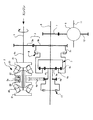•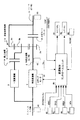•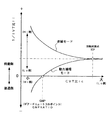•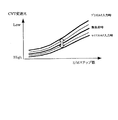•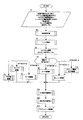•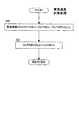••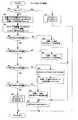••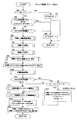•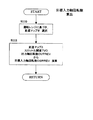•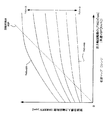•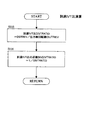•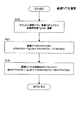•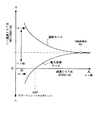•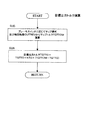•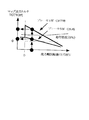•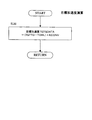•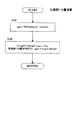•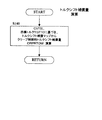•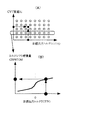•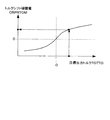•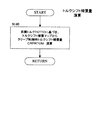••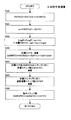•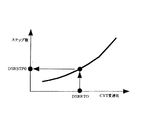•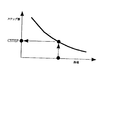•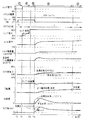•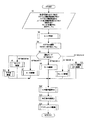•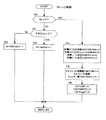•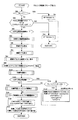•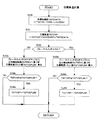•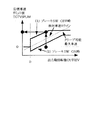•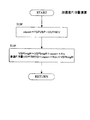•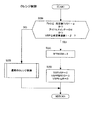•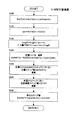•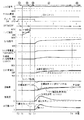•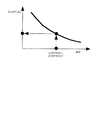•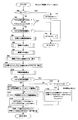•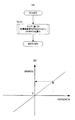•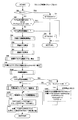•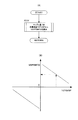## Classifications

• FMECHANICAL ENGINEERING; LIGHTING; HEATING; WEAPONS; BLASTING
• F16ENGINEERING ELEMENTS AND UNITS; GENERAL MEASURES FOR PRODUCING AND MAINTAINING EFFECTIVE FUNCTIONING OF MACHINES OR INSTALLATIONS; THERMAL INSULATION IN GENERAL
• F16HGEARING
• F16H61/00Control functions within control units of change-speed- or reversing-gearings for conveying rotary motion ; Control of exclusively fluid gearing, friction gearing, gearings with endless flexible members or other particular types of gearing
• F16H61/66Control functions within control units of change-speed- or reversing-gearings for conveying rotary motion ; Control of exclusively fluid gearing, friction gearing, gearings with endless flexible members or other particular types of gearing specially adapted for continuously variable gearings
• F16H61/664Friction gearings
• F16H61/6648Friction gearings controlling of shifting being influenced by a signal derived from the engine and the main coupling
• FMECHANICAL ENGINEERING; LIGHTING; HEATING; WEAPONS; BLASTING
• F16ENGINEERING ELEMENTS AND UNITS; GENERAL MEASURES FOR PRODUCING AND MAINTAINING EFFECTIVE FUNCTIONING OF MACHINES OR INSTALLATIONS; THERMAL INSULATION IN GENERAL
• F16HGEARING
• F16H37/00Combinations of mechanical gearings, not hereinbefore provided for
• F16H37/02Combinations of mechanical gearings, not hereinbefore provided for comprising essentially only toothed or friction gearings
• F16H37/06Combinations of mechanical gearings, not hereinbefore provided for comprising essentially only toothed or friction gearings with a plurality of driving or driven shafts; with arrangements for dividing torque between two or more intermediate shafts
• F16H37/08Combinations of mechanical gearings, not hereinbefore provided for comprising essentially only toothed or friction gearings with a plurality of driving or driven shafts; with arrangements for dividing torque between two or more intermediate shafts with differential gearing
• F16H37/0833Combinations of mechanical gearings, not hereinbefore provided for comprising essentially only toothed or friction gearings with a plurality of driving or driven shafts; with arrangements for dividing torque between two or more intermediate shafts with differential gearing with arrangements for dividing torque between two or more intermediate shafts, i.e. with two or more internal power paths
• F16H37/084Combinations of mechanical gearings, not hereinbefore provided for comprising essentially only toothed or friction gearings with a plurality of driving or driven shafts; with arrangements for dividing torque between two or more intermediate shafts with differential gearing with arrangements for dividing torque between two or more intermediate shafts, i.e. with two or more internal power paths at least one power path being a continuously variable transmission, i.e. CVT
• F16H2037/088Power split variators with summing differentials, with the input of the CVT connected or connectable to the input shaft
• FMECHANICAL ENGINEERING; LIGHTING; HEATING; WEAPONS; BLASTING
• F16ENGINEERING ELEMENTS AND UNITS; GENERAL MEASURES FOR PRODUCING AND MAINTAINING EFFECTIVE FUNCTIONING OF MACHINES OR INSTALLATIONS; THERMAL INSULATION IN GENERAL
• F16HGEARING
• F16H37/00Combinations of mechanical gearings, not hereinbefore provided for
• F16H37/02Combinations of mechanical gearings, not hereinbefore provided for comprising essentially only toothed or friction gearings
• F16H37/06Combinations of mechanical gearings, not hereinbefore provided for comprising essentially only toothed or friction gearings with a plurality of driving or driven shafts; with arrangements for dividing torque between two or more intermediate shafts
• F16H37/08Combinations of mechanical gearings, not hereinbefore provided for comprising essentially only toothed or friction gearings with a plurality of driving or driven shafts; with arrangements for dividing torque between two or more intermediate shafts with differential gearing
• F16H37/0833Combinations of mechanical gearings, not hereinbefore provided for comprising essentially only toothed or friction gearings with a plurality of driving or driven shafts; with arrangements for dividing torque between two or more intermediate shafts with differential gearing with arrangements for dividing torque between two or more intermediate shafts, i.e. with two or more internal power paths
• F16H37/084Combinations of mechanical gearings, not hereinbefore provided for comprising essentially only toothed or friction gearings with a plurality of driving or driven shafts; with arrangements for dividing torque between two or more intermediate shafts with differential gearing with arrangements for dividing torque between two or more intermediate shafts, i.e. with two or more internal power paths at least one power path being a continuously variable transmission, i.e. CVT
• F16H37/086CVT using two coaxial friction members cooperating with at least one intermediate friction member
• YGENERAL TAGGING OF NEW TECHNOLOGICAL DEVELOPMENTS; GENERAL TAGGING OF CROSS-SECTIONAL TECHNOLOGIES SPANNING OVER SEVERAL SECTIONS OF THE IPC; TECHNICAL SUBJECTS COVERED BY FORMER USPC CROSS-REFERENCE ART COLLECTIONS [XRACs] AND DIGESTS
• Y10TECHNICAL SUBJECTS COVERED BY FORMER USPC
• Y10STECHNICAL SUBJECTS COVERED BY FORMER USPC CROSS-REFERENCE ART COLLECTIONS [XRACs] AND DIGESTS
• Y10S477/00Interrelated power delivery controls, including engine control
• Y10S477/904Control signal is acceleration
• YGENERAL TAGGING OF NEW TECHNOLOGICAL DEVELOPMENTS; GENERAL TAGGING OF CROSS-SECTIONAL TECHNOLOGIES SPANNING OVER SEVERAL SECTIONS OF THE IPC; TECHNICAL SUBJECTS COVERED BY FORMER USPC CROSS-REFERENCE ART COLLECTIONS [XRACs] AND DIGESTS
• Y10TECHNICAL SUBJECTS COVERED BY FORMER USPC
• Y10TTECHNICAL SUBJECTS COVERED BY FORMER US CLASSIFICATION
• Y10T477/00Interrelated power delivery controls, including engine control
• Y10T477/60Transmission control
• Y10T477/619Continuously variable friction transmission
• YGENERAL TAGGING OF NEW TECHNOLOGICAL DEVELOPMENTS; GENERAL TAGGING OF CROSS-SECTIONAL TECHNOLOGIES SPANNING OVER SEVERAL SECTIONS OF THE IPC; TECHNICAL SUBJECTS COVERED BY FORMER USPC CROSS-REFERENCE ART COLLECTIONS [XRACs] AND DIGESTS
• Y10TECHNICAL SUBJECTS COVERED BY FORMER USPC
• Y10TTECHNICAL SUBJECTS COVERED BY FORMER US CLASSIFICATION
• Y10T477/00Interrelated power delivery controls, including engine control
• Y10T477/60Transmission control
• Y10T477/688Transmission controlled by engine
• Y10T477/692Transmission controlled by engine by acceleration

## Description


BACKGROUND OF THE INVENTION
The present invention relates to an improvement in a control device for an infinitely variable gear ratio continuously variable transmission employed in a vehicle or the like.

[Prior art]
2. Description of the Related Art Conventionally, as a transmission of a vehicle, a continuously variable transmission with an infinite gear ratio that can control the gear ratio to infinity is known. For example, Japanese Patent Application Laid-Open No. 2000-179664 discloses a gear ratio of infinity. In creep start / stop from the geared neutral point (GNP position), the speed ratio of the continuously variable transmission mechanism is changed by controlling the step motor position to achieve the target vehicle speed.

In this case, when the brake SW is OFF and the accelerator is released in the forward travel range, the control is performed so that a predetermined vehicle speed (corrected according to the gradient and the steering angle) is obtained.

[Problems to be solved by the invention]
By the way, a conventional vehicle with an automatic transmission generally generates an appropriate creep torque (driving force) when starting and stopping, thereby realizing a smooth driving feeling.

However, the vehicle speed itself is determined by the creep torque (driving force) to be generated, the vehicle weight, etc., but in the conventional example, the vehicle speed is simply given as the target value. Even if the brake is on (braking state), even if the brake is off, the target vehicle speed will be achieved, so the generated creep torque will be different from what the driver expects, giving a sense of incongruity There was a problem.

Accordingly, the present invention has been made in view of the above problems, and an object of the present invention is to generate creep torque as expected by the driver when a continuously variable transmission with an infinite gear ratio is used.

[Means for Solving the Problems]
According to a first aspect of the present invention, a toroidal continuously variable transmission mechanism and a constant transmission mechanism capable of continuously changing a gear ratio are connected to a unit input shaft, respectively, and an output shaft of the continuously variable transmission mechanism and the constant transmission mechanism is connected to the unit input shaft. An infinitely variable gear ratio continuously variable transmission connected to the unit output shaft via a planetary gear mechanism, a power circulation mode clutch and a direct coupling mode clutch, and the total gear ratio or the gear ratio of the continuously variable transmission mechanism is controlled according to the operating state. In a control device for a continuously variable transmission with an infinite gear ratio comprising a gear ratio control means,
Accelerator operation amount detection means for detecting the accelerator operation amount, brake state detection means for detecting the brake operation state, and target drive force calculation means for calculating the target drive force from the driving state including the accelerator operation amount or the brake operation state Target acceleration calculating means for calculating target acceleration based on the target driving force and driving state, range detecting means for detecting a driving range based on driving operation, vehicle speed detecting means for detecting vehicle speed, and at least the driving Creep control determination means for determining execution of creep control based on a driving state including a range, a vehicle speed, and an accelerator operation amount; target IVT ratio calculation means for calculating a target total gear ratio based on the driving state of the vehicle; Target CVT ratio calculating means for calculating the target speed ratio of the continuously variable transmission mechanism based on the target total speed ratio, and the target speed ratio Correction means for correcting by feedback control based on a deviation between acceleration and the target acceleration, and the speed ratio control means becomes the corrected target speed ratio when it is determined that the creep control is to be executed. In this way, the gear ratio is controlled to generate creep.

According to a second aspect of the present invention, a toroidal continuously variable transmission mechanism and a constant transmission mechanism capable of continuously changing the transmission gear ratio are connected to the unit input shaft, respectively, and outputs of the continuously variable transmission mechanism and the constant transmission mechanism are provided. An infinite gear ratio continuously variable transmission whose shaft is connected to the unit output shaft via a planetary gear mechanism, a power circulation mode clutch and a direct coupling mode clutch, and the total gear ratio or the gear ratio of the continuously variable transmission mechanism depending on the operating state. In a control device for an infinitely variable gear ratio continuously variable transmission comprising a gear ratio control means for controlling,
Accelerator operation amount detection means for detecting the accelerator operation amount, brake state detection means for detecting the brake operation state, and target drive force calculation means for calculating the target drive force from the driving state including the accelerator operation amount or the brake operation state Target acceleration calculating means for calculating target acceleration based on the target driving force and driving state, range detecting means for detecting a driving range based on driving operation, vehicle speed detecting means for detecting vehicle speed, and at least the driving Creep control determination means for determining execution of creep control based on a driving state including a range, a vehicle speed, and an accelerator operation amount; target IVT ratio calculation means for calculating a target total gear ratio based on the driving state of the vehicle; A target CVT ratio calculating means for calculating a target speed ratio of the continuously variable transmission mechanism based on the target total speed ratio, Correction means for correcting by feedforward control based on the target acceleration, and when the execution of the creep control is determined, the speed ratio control means adjusts the speed ratio so that the corrected target speed ratio is obtained. Control to generate creep.

According to a third aspect of the present invention, a toroidal continuously variable transmission mechanism and a constant transmission mechanism capable of continuously changing a gear ratio are connected to a unit input shaft, respectively, and outputs of the continuously variable transmission mechanism and the constant transmission mechanism are provided. An infinite gear ratio continuously variable transmission whose shaft is connected to the unit output shaft via a planetary gear mechanism, a power circulation mode clutch and a direct coupling mode clutch, and the total gear ratio or the gear ratio of the continuously variable transmission mechanism depending on the operating state. In a control device for an infinitely variable gear ratio continuously variable transmission comprising a gear ratio control means for controlling,
Accelerator operation amount detection means for detecting the accelerator operation amount, brake state detection means for detecting the brake operation state, and target drive force calculation means for calculating the target drive force from the driving state including the accelerator operation amount or the brake operation state A target acceleration calculating means for calculating a target acceleration based on the target driving force and the driving state, a target vehicle speed calculating means for calculating a target vehicle speed by time integration of the target acceleration, and a driving range based on the driving operation. Range detection means for detecting vehicle speed, vehicle speed detection means for detecting vehicle speed, creep control determination means for determining execution of creep control based on at least the driving range, vehicle speed, and accelerator operation amount, and vehicle driving state Target IVT ratio calculating means for calculating a target total transmission ratio based on the target total transmission ratio, and continuously variable transmission based on the target total transmission ratio A target CVT ratio calculating means for calculating a target gear ratio, a correcting means for correcting the target gear ratio by feedback control based on a deviation between an actual vehicle speed and the target vehicle speed, and the gear ratio control means, When it is determined that creep control is to be executed, creep is generated by controlling the gear ratio so that the corrected target gear ratio is obtained.

According to a fourth aspect of the present invention, a toroidal continuously variable transmission mechanism and a constant transmission mechanism capable of continuously changing the transmission gear ratio are connected to the unit input shaft, respectively, and outputs of the continuously variable transmission mechanism and the constant transmission mechanism are provided. An infinite gear ratio continuously variable transmission whose shaft is connected to the unit output shaft via a planetary gear mechanism, a power circulation mode clutch and a direct coupling mode clutch, and the total gear ratio or the gear ratio of the continuously variable transmission mechanism depending on the operating state. In a control device for an infinitely variable gear ratio continuously variable transmission comprising a gear ratio control means for controlling,
Accelerator operation amount detection means for detecting the accelerator operation amount, brake state detection means for detecting the brake operation state, and target drive force calculation means for calculating the target drive force from the driving state including the accelerator operation amount or the brake operation state A target acceleration calculating means for calculating a target acceleration based on the target driving force and the driving state, a target vehicle speed calculating means for calculating a target vehicle speed by time integration of the target acceleration, and a driving range based on the driving operation. Range detection means for detecting vehicle speed, vehicle speed detection means for detecting vehicle speed, creep control determination means for determining execution of creep control based on at least the driving range, vehicle speed, and accelerator operation amount, and vehicle driving state Target IVT ratio calculating means for calculating a target total transmission ratio based on the target total transmission ratio, and continuously variable transmission based on the target total transmission ratio Target CVT ratio calculating means for calculating the target gear ratio, correction means for correcting the target gear ratio by feedforward control based on the target vehicle speed, and the gear ratio control means determine whether the creep control is executed. When this is done, creep is generated by controlling the gear ratio so that the corrected target gear ratio is obtained.

In a fifth aspect based on any one of the first to fourth aspects, the target driving force calculation means calculates a target driving force based on a brake operation state and a vehicle speed or a vehicle speed equivalent value. .

In a sixth aspect based on any one of the first to fifth aspects, the target CVT ratio calculation means is a target of the continuously variable transmission mechanism according to a torque shift compensation amount based on the target driving force. Correct the gear ratio.

【The invention's effect】
Therefore, according to the first aspect of the present invention, when the driving state becomes a preset creep control state, the target driving force (target output torque) is calculated from the driving state of the vehicle including the accelerator operation and the brake operation of the driver. Since the target gear ratio (target CVT ratio) is corrected by feedback control based on the deviation between the target acceleration calculated from the driving force and the actual acceleration, the creep torque and vehicle acceleration that meet the driver's expectations are achieved. It is possible to improve the drivability of a vehicle equipped with a continuously variable transmission with an infinite gear ratio.

The second aspect of the invention calculates a target driving force (target output torque) from the driving state of the vehicle including the accelerator operation and the brake operation of the driver when the driving state becomes a preset creep control state. Since the target gear ratio (target CVT ratio) is corrected by feedforward control based on the target acceleration calculated from the driving force, creep torque and vehicle acceleration that meet the driver's expectations can be realized, and the gear ratio is infinite. The drivability of a vehicle equipped with a continuously variable transmission can be improved.

In the third invention, when the driving state becomes a preset creep control state, the target driving force (target output torque) and the target ideal acceleration are calculated from the driving state of the vehicle including the accelerator and brake operation of the driver. Since the target gear ratio (target CVT ratio) is corrected by feedback control based on the deviation between the target vehicle speed calculated by integrating the target acceleration and the actual vehicle speed, creep that meets the driver's expectations Torque and vehicle speed can be realized, and the drivability of a vehicle equipped with an infinitely variable gear ratio continuously variable transmission can be improved.

According to a fourth aspect of the present invention, when the driving state becomes a preset creep control state, the target driving force (target output torque) and the target ideal acceleration are calculated from the driving state of the vehicle including the driver's accelerator and brake operations. Because the target gear ratio (target CVT ratio) is corrected by feedforward control based on the target vehicle speed calculated by integrating the target acceleration, the creep torque and vehicle speed that meet the driver's expectations are realized. It is possible to improve the drivability of a vehicle equipped with a continuously variable transmission with an infinite gear ratio.

In the fifth aspect of the invention, the target driving force corresponds to the brake operation state (ON / OFF) and is calculated based on the vehicle speed equivalent value such as the output shaft rotation speed. Can be along.

In the sixth aspect of the invention, since the target gear ratio is corrected by the torque shift amount in accordance with the target driving force, the creep torque (and vehicle acceleration and vehicle speed) can be obtained with higher accuracy.

DETAILED DESCRIPTION OF THE INVENTION
Hereinafter, an embodiment of the present invention will be described with reference to the accompanying drawings.

As shown in FIGS. 1 and 2, the infinitely variable gear ratio continuously variable transmission includes a toroidal continuously variable transmission mechanism 2 capable of continuously changing a gear ratio to a unit input shaft 1 connected to an engine, The constant speed change mechanism 3 (reduction gear) composed of the gear 3a and the gear 3b is connected in parallel, and the output shafts 4 and 3c are coaxially arranged on the unit output shaft 6 and connected on the planetary gear mechanism 5. Thus, the output shaft 4 of the continuously variable transmission mechanism 2 is connected to the sun gear 5a and the direct coupling mode clutch 10 of the planetary gear mechanism 5, and the output shaft 3c of the constant transmission mechanism 3 is connected to the planetary gear via the power circulation mode clutch 9. The gear mechanism 5 is connected to the carrier 5b.

The continuously variable transmission output shaft 4 connected to the sun gear 5a transmits the driving force to the continuously variable transmission mechanism 2 from the sprocket 4a and the chain 4b, and the infinite gear ratio is continuously variable via the direct coupling mode clutch 10. Is selectively coupled to the unit output shaft 6 which is the output shaft of the machine.

On the other hand, the carrier 5b selectively coupled to the output shaft 3c of the constant speed change mechanism 3 via the power circulation mode clutch 9 is connected to the unit output shaft 6 via the ring gear 5c.

The unit output shaft 6 is provided with a transmission output gear 7. The transmission output gear 7 meshes with the final gear 12 of the differential gear 8, and the drive shaft 11 coupled to the differential gear 8 has a predetermined total reduction ratio. The driving force is transmitted at (IVT ratio ii = unit input shaft rotational speed / unit output shaft rotational speed).

As shown in FIG. 1, the continuously variable transmission mechanism 2 is composed of a double-cavity half-toroidal type that holds and presses the power rollers 20 and 20 with two sets of an input disk 21 and an output disk 22, and a pair of outputs. The output sprocket 2a interposed between the disks 22 is connected to a sprocket 4a formed on the continuously variable transmission output shaft 4 of the unit output shaft 6 disposed in parallel with the unit input shaft 1 via a chain 4b. .

The unit input shaft 1 and the CVT shaft 1b are coupled in a rotational direction via a loading cam device (not shown). The unit input shaft 1 is coupled to the engine and forms a gear 3a of the constant speed change mechanism 3. The CVT shaft 1b is connected to two sets of input disks 21 and 21, and the power rollers 20 and 20 are inserted by the axial pressing force generated by the loading cam device according to the input torque from the unit input shaft 1. Clamp and press between output disks.

In this infinitely variable transmission continuously variable transmission, as shown in FIG. 3, the power circulation mode clutch 9 is disengaged, and the direct coupling mode clutch 10 is engaged and the gear ratio ic (hereinafter referred to as CVT ratio) of the continuously variable transmission mechanism 2. ic) and the power circulation mode clutch 9 are engaged, while the direct connection mode clutch 10 is released, whereby the transmission ratio of the continuously variable transmission mechanism 2 and the constant transmission mechanism 3 is determined. Depending on the difference, the total gear ratio of the entire continuously variable transmission with infinite gear ratio (the gear ratio of the unit input shaft 1 and the unit output shaft 6, hereinafter referred to as IVT ratio ii) is changed from a negative value to a positive value. It is possible to selectively use a power circulation mode in which control is performed almost continuously including infinity.

Then, at the geared neutral point GNP in the power circulation mode in which the IVT ratio ii is infinite (in FIG. 3, the reciprocal 1 / ii = 0 of the IVT ratio ii), the vehicle can be stopped while being connected from the engine to the drive shaft. The vehicle can be started by changing the IVT ratio ii from this stop state.

Further, for example, as shown in FIG. 3, the switching between the power circulation mode and the direct connection mode is a rotation synchronization point where the rotation speeds of the output shaft 4 of the continuously variable transmission mechanism 2 and the output shaft 3c of the constant transmission mechanism 3 coincide. If the operation is performed in the vicinity of the RSP as described later, the operation mode can be smoothly switched without causing a switching shock.

Note that the rotation synchronization point RSP is a point at which the IVT ratio matches at the same CVT ratio in the power circulation mode and the direct connection mode.

Here, the CVT ratio ic of the toroidal-type continuously variable transmission mechanism 2 is controlled with the characteristics shown in FIG. 4 by controlling the number of steps of the step motor 36 shown in FIG.

Here, FIG. 4 is a map in which the relationship between the CVT ratio of the toroidal-type continuously variable transmission mechanism 2 and the number of steps is set with the input torque as a parameter. As is known as a toroidal-type characteristic, This shows the influence of torque shift in which the CVT ratio changes accordingly.

That is, if the input torque to the continuously variable transmission mechanism 2 increases in the positive direction, the CVT ratio moves to the low side, and if the input torque increases in the negative direction, the CVT ratio moves to the high side.

On the other hand, in the continuously variable transmission with an infinite gear ratio, the direction of the torque passing through the continuously variable transmission mechanism 2 changes according to the operation mode, and the torque is transmitted from the output disk 22 to the input disk 21 when the power circulation mode advances. In the negative direction to be transmitted, the torque is transmitted from the input disk 21 to the output disk 22 in the reverse direction in the power circulation mode and in the direct connection mode.

Accordingly, when the input torque increases during forward movement in the power circulation mode, the passing torque of the continuously variable transmission mechanism 2 increases in the negative direction, so that the CVT ratio moves to the high side, and thereby the IVT ratio changes to the low side. . In order to compensate for this torque shift, a predetermined speed ratio can be maintained by performing speed change control in a direction opposite to the direction in which the CVT ratio and the IVT ratio change.

The clutch that switches between the power circulation mode and the direct connection mode is controlled by a direct connection solenoid 91 that controls the direct connection mode clutch 10 and a power circulation solenoid 92 that controls the power circulation mode clutch 9.

Next, FIG. 2 shows a block diagram including a control system of an infinitely variable transmission continuously variable transmission.

The shift control control unit 80 mainly composed of a microcomputer includes an output from the input shaft rotational speed sensor 81 for detecting the rotational speed INPREV of the unit input shaft 1, that is, the engine rotational speed Ne, and an output of the continuously variable transmission mechanism 2. The output from the output shaft speed sensor 82 for detecting the shaft speed No, the output from the vehicle speed sensor 83 for detecting the rotational speed OUTREV of the unit output shaft 6 as the vehicle speed VSP, and the throttle opening TVO (or the depression amount of the accelerator pedal) Output from the accelerator operation amount sensor 84 for detecting APS), output from the inhibitor switch 85 for detecting the select position RNG according to the operation of the select switch, an ON / OFF signal from the brake switch 86 that responds to the brake pedal, Idles that detect the release state of the accelerator pedal ON / OFF signal pitch 87 is detected, the output or the like of the oil temperature sensor 88 for detecting the oil temperature TEMP of IVT are input.

The vehicle speed VSP is calculated by multiplying the rotational speed OUTREV of the unit output shaft 6 by a predetermined constant.

The select position RNG includes, for example, a D range (forward range), an R range (reverse range), an N range (neutral range), and an L range (low range).

The shift control control unit 80 processes the detection values of these various sensors as operating states, obtains the ultimate input shaft rotational speed DSRREV from a preset shift map based on the throttle opening TVO and the vehicle speed VSP, and outputs this as the unit output. By dividing the shaft rotational speed OUTREV to determine the reached IVT ratio DIVTRATIO, the CVT ratio ic is controlled, and when the operation mode needs to be switched, the power circulation mode clutch 9 and the direct connection mode clutch 10 are selectively selected. Fasten and switch between power circulation mode and direct coupling mode.

Here, an example of the control performed by the shift control control unit 80 will be described in detail below with reference to the flowcharts of FIGS. This control is executed every predetermined time, for example, every 10 msec.

First, the flowchart of FIG. 5 shows the main routine of the shift control. In step S1, the unit input shaft rotational speed INPREV, the continuously variable transmission output shaft rotational speed No, the unit output shaft rotational speed OUTREV ( Vehicle speed VSP), select position RNG, signal from brake switch 86, detection of hydraulic pressures Plo and Phi of a hydraulic cylinder (not shown) for instructing trunnion of continuously variable transmission mechanism 2 and various detection states indicating throttle opening TVO Read the value.

Next, in step S2, the actual acceleration GDATA generated in the vehicle is detected. The actual acceleration GDATA is obtained by multiplying the difference between the current output shaft rotational speed OUTREV and the previous output shaft rotational speed OUTREVOLD (10 msec before) by a predetermined constant KG as in steps S20 and S21 of FIG. 6 (step S20). ) Substituting the current output shaft rotational speed OUTREV into the previous value OUTREVOL to prepare for the next control.

In step S3, based on the detected select position RNG, it is determined which operating range is present.

In step S4, the actual CVT ratio RATIO is calculated from the ratio (INPREV / No) of the detected unit input shaft rotational speed INPREV and continuously variable transmission mechanism output shaft rotational speed No.

In step S5, based on each detection value, it is determined which of the following operation modes SFTMODE is. If the N range (SFTMODE = 0), the process proceeds to the N range control in step S6. If D select (SFTMODE = 1), the process proceeds to ND control in step S7. If DN select (SFTMODE = 2), the process proceeds to DN control in step S8. If it is in the creep operation region (SFTMODE = 3), the process proceeds to D range control in step S9. If it is in the power circulation mode of the D range and is not in the creep operation region (SFTMODE = 4), the process proceeds to control in step S10. In the direct connection mode, the R range, etc., the process proceeds to other control in step S11.

Then, after performing the process according to the operation mode SFTMODE, the process proceeds to step S12 to calculate the number of steps DSRSTP of the step motor 36 according to the target value (described later), and in step S13, from step S6 to step S11 From the calculated target hydraulic pressure values DSRPRSLC and DSRPRSHC of the power circulation mode clutch and the direct connection mode clutch, a hydraulic pressure command value (for example, a duty ratio) to be supplied to the solenoids 91 and 92 of the power circulation mode clutch 9 or the direct connection mode clutch 10 is obtained ( (See FIG. 38).

Based on these step number DSRSTP and the hydraulic pressure command value, in step S14, the step motor 36 and the direct connection solenoid 91 or the power circulation solenoid 92 are driven.

Next, the N range control performed in step S6 will be described with reference to the subroutine of FIG.

First, in step S40, it is determined whether the detected select position RNG is in the N range. If it is not in the N range, the process branches to step S41, and the forward range (D or L range) in which the D range or L range is selected. It is judged whether it is.

If it is the forward range, the process branches to step S42 to set SFTMODE = 1 (N → D select control). Otherwise, this routine is ended with SFTMODE = −1 (N → R select control).

On the other hand, when the determination in step S40 is the N range, the process proceeds to step S44, where the reciprocal of the target IVT ratio INVIVTRATIO0 = 0, the target speed ratio RATIO0 = GNPRATIO (fixed value corresponding to GNP) of the continuously variable transmission mechanism 2, and the IVT ratio. Is set to the geared neutral point GNP position.

Further, in the N range, the power circulation mode and the direct coupling mode clutches 9 and 10 are both released, so that the respective target oil pressures DSRPRSLC and DSRPRSHC = 0.

In step S45, since there is no need for torque shift compensation in the N range, the torque shift compensation amount TSRTOM = 0 and the torque shift compensation amount filter value (primary filter value) TSRTOMFL = 0.

In step S46, in preparation for acceleration F / B control during creep control, the gear ratio compensation amount GFBRTO = 0 of the continuously variable transmission mechanism 2 by acceleration F / B and the integral compensation amount GIntgR = 0 by acceleration F / B are set.

Next, the N → D select control will be described with reference to FIG.

First, in step S50, it is determined whether the detected select position RNG is in the N range.

If it is the N range, the process branches to step S51, where the variable INTGND = 0 and the timer NDTIMER = 0, the N → D select control is ended, and the operation mode SFTMODE = 2 is set, and the D → N control is started next time.

If it is not the N range, the process proceeds to step S52, and the reciprocal of the target IVT ratio INVIVTRATIO0 = 0 and the target speed ratio RATIO0 = GNPRATIO of the continuously variable transmission mechanism 2 are set so that the IVT ratio becomes the geared neutral point GNP position.

Further, since the direct connection mode clutch 10 is being released, the target hydraulic pressure DSRPRSHC = 0 is set.

In step S53, since there is no need for torque shift compensation in the N range, the torque shift compensation amount TSRTOM = 0 and the filter value TSRTOMFL = 0 are set.

In steps S54 to S64, sequence control by a timer is performed in order to engage the power circulation mode clutch 9 from the released state.

That is, in step S63, the selection timer NDTIMER is sequentially incremented, and branch destinations 58 to 64 are selected in steps S54 to 57 according to the progress.

In step S54, when the timer NDTIMER is less than the predetermined value 1, the target oil pressure DSRPRSLC of the power circulation mode clutch 9 is set to the ND predetermined value 1 (precharge pressure).

Next, in the determination in step S55, in step S59 where the timer NDTIMER is equal to or greater than the predetermined value 1 and less than the predetermined value 2, the target hydraulic pressure DSRPRSLC = ND predetermined value 2 (precharge pressure 2) is inserted into the power circulation mode clutch 9. I do.

Next, in step S56, in step S60 where the timer NDTIMER is greater than or equal to the predetermined value 2 and less than the predetermined value 3, the target hydraulic pressure is increased.
INTGND = INTGND + DELETE
In step S61,
Target oil pressure DSRPRSLC = ND predetermined value 2 + INTGND
The ramp control is performed to increase the target hydraulic pressure, and the power circulation mode clutch 9 is gradually engaged.

Next, in step S57 in which the timer NDTIMER is greater than or equal to the predetermined value 3 and less than the predetermined value 4 in step S57, the power circulation mode clutch 9 is completely engaged with the target oil pressure DSRPRSLC = the oil pressure maximum value.

Then, when the timer NDTIMER has passed the predetermined time 4 in the determination of step S57, the process proceeds to step S64 where the power circulation mode clutch 9 is engaged and shifts to the D range, and the ND selection is performed with the variable INTGND = 0 and the timer NDTIMER = 0. The control is ended, and the D range dynamic circulation (power circulation mode) control is started from the next time with the operation mode SFTMODE = 3.

Next, the D → N select control will be described with reference to FIG.

First, in step S70, it is determined whether the detected select position RNG is in the D range.

If it is in the D range, the process branches to step S71, and the D → N select control is terminated with the variable INTGDN = 0 and the timer DNTIMER = 0, and the N → D control is started next time with the operation mode SFTMODE = 1.

On the other hand, if it is determined in step S70 that it is not in the D range, the process proceeds to step S72, and the reciprocal of the target IVT ratio INVIVTRATIO0 = 0 and the target transmission ratio RATIO0 = GNPRATIO of the continuously variable transmission mechanism 2 are set.

Further, in order to release the direct connection mode clutch 10 in the N range, this target hydraulic pressure DSRPRSHC = 0.

In step S73, since there is no need for torque shift compensation in the N range, the torque shift compensation amount TSRTOM = 0 and the filter value TSRTOMFL = 0 are set.

In steps S74 to S80, a sequence control by a timer is performed to release the power circulation mode clutch 9 from the engaged state.

That is, in step S79, the selection timer DNTIMER is sequentially incremented, and branch destinations 76 to 80 are selected in steps S74 and S75 according to the progress.

In step S74, while the timer DNTIMER is less than the predetermined value 1, in step S76, the target hydraulic pressure of the power circulation mode clutch 9 is set.
In step S77, the target hydraulic pressure DSRPRSLC of the power circulation mode clutch 9 is
DSRPRSLC = DN predetermined value 1 + INTGDN
The power circulation mode clutch 9 is gradually released by gradually reducing the pressure by ramp control. However, the minimum value of the target hydraulic pressure DSRPRSLC is restricted to 0.

Next, when the timer DNTIMER is greater than or equal to the predetermined value 1 and less than the predetermined value 2 in the determination in step S75, the target hydraulic pressure DSRPRSLC = 0 is set in step S78 and the power circulation mode clutch 9 is completely released.

Then, when the predetermined value 2 has elapsed in the determination of step S75, the process proceeds to step S80 to finish the DN select control with INTGDN = 0 and DNTIMER = 0, and start the N range control with the operation mode SFTMODE = 0 from the next time. .

Next, processing in D range dynamic circulation (power circulation mode) control will be described based on FIG.

Here, the main part of the present invention is shown, and acceleration F / B is performed by creep control.

First, in step S90, it is determined whether the detected select position RNG is in the N range.

If it is the N range, the process branches to step S91 to set the operation mode SFTMODE = 2, and the D → N control is started from the next time.

On the other hand, if it is not the N range, the process branches to step S92.

In steps S92 and S93, it is determined whether or not the creep control region has shifted to normal running (no creep) in the power circulation mode.

That is, in step S92, it is determined whether the throttle opening TVO ≧ predetermined value TVO1 or the idle SW is OFF, and then in step S93, it is determined whether vehicle speed VSP ≧ predetermined vehicle speed value 1 is satisfied. If any of these conditions is satisfied, the process branches to step S94. In step S94, the operation mode SFTMODE = 4 is set and normal running in the D range dynamic circulation mode is started from the next time.

On the other hand, when the conditions of steps S92 and 93 are not satisfied, the process proceeds to step S95 and thereafter, and creep control is performed.

In step S95, the target input rotational speed DSRREV is calculated according to the subroutine of FIG.

That is, in FIG. 11, in step S110, a shift map corresponding to the detected select position RNG is selected. In this case, since it is the D range, a shift map of the D range as shown in FIG. 12 is selected.

Next, in step S111, the target target rotational speed DSRREV is calculated from the selected shift map based on the throttle opening TVO and the output shaft rotational speed OUTREV by table search (a method used in general CVT or the like). the same as).

Next, in step S96 of FIG. 10, the reached IVT ratio DIVTRATIO and its inverse INVDIVTRATIO are calculated according to the subroutine of FIG.

That is, in the subroutine of FIG. 13, the target IVT speed DSRREV is divided by the output shaft speed OUTREV in step S115 to calculate the target IVT gear ratio DIVTRATIO. calculate.

Next, in step S97 of FIG. 10, the target IVT ratio IVTRATIO0 and its inverse INVIVTRATIO0 at the time of transition are calculated according to the subroutine of FIG.

That is, in FIG. 14, in step S120, a shift time constant TgTM for calculating the speed ratio at the transition of IVT is calculated from parameters such as the throttle opening TVO and the vehicle speed VSP.

Next, in step S121, a transient IVT gear ratio IVTRATIO0 is calculated based on the following equation.

Next, in step S122, a reciprocal number INVIVTRATIO0 of the transient IVT gear ratio is calculated based on the following equation.

The formula used in steps S121 and S122 represents a general low-pass filter. This is for creating a target value at the time of transition, and may have a configuration such as a second-order lag filter, which is appropriately changed according to the purpose of IVT.

Next, in step S98 in FIG. 10, a map search is performed from the reciprocal number INVIVTRATIO0 of the target IVT ratio at the time of transition according to the map of FIG. 15 to calculate the target CVT ratio RATIO0 at the time of transition of the continuously variable transmission mechanism 2.

In step S99, the target output torque at the time of creep control is calculated according to the subroutine of FIG.

That is, in step S125 of FIG. 16, a characteristic map corresponding to ON / OFF of the brake switch 86 is selected in the map of FIG. 17, a map search is performed based on the output shaft rotational speed OUTREV, and the map output torque TGTTOM is calculated. calculate.

This characteristic map is set so that the map output torque increases as the output shaft speed decreases, and is set so that a sufficiently larger torque than the running resistance of the vehicle (equivalent to a flat road) can be obtained when the brake is off. And make sure the vehicle moves forward.

Further, when the brake is ON, the map output torque is set to be smaller than the running resistance, and appropriate deceleration is given.

Next, in step S126, the target output torque TGTTO is calculated by the following equation. TGTTO = TGTTO + KTO × (TGTTTOM-TGTTO)
This equation is a so-called low-pass filter, and KTO indicates its time constant. By adding this low-pass filter, the target torque changes smoothly even when the brake is repeatedly turned on and off, thereby preventing deterioration of drivability during F / B control.

Next, in step S100 of FIG. 10, the target acceleration TGTGDATA corresponding to the target output torque TGTTO is calculated according to the subroutine of FIG.

That is, in step S130 of FIG. 18, the target acceleration TGTGDATA is calculated by the following equation.

TGTGDATA = (TGTTO-TORL) × KCONV
Here, TORL is a value indicating the running resistance of the vehicle, and includes a windage loss, a gradient, etc. according to the vehicle speed VSP. The constant KCONV is a conversion coefficient including information such as vehicle weight and tire diameter.

Next, in step S101 of FIG. 10, it is determined whether vehicle speed VSP ≦ predetermined vehicle speed value 2.

If the vehicle speed value is less than or equal to 2, the process branches to step S102 to determine whether or not the brake switch 86 is ON. If it is ON, it is determined that the vehicle is almost stopped and the process proceeds to step S104.

In step S104, the reciprocal of the target IVT ratio INVIVTRATIO0 = 0 and the target speed ratio RATIO0 = GNPRATIO of the continuously variable transmission mechanism 2 are set for the purpose of keeping the vehicle stopped at the geared neutral point GNP position. Further, the acceleration feedback compensation amount GFBRTO = 0 and the integral compensation amount GIntgR = 0 are set in preparation for the restart of the creep control.

On the other hand, if the vehicle speed is greater than or equal to the predetermined value 2 in step S101 or the brake switch 86 is OFF in step S102, it is determined that creep travel is to continue and branch to step S103 to calculate the feedback amount GFBRTO based on the target acceleration TGTGDATA. . In step S103, feedback calculation based on the target acceleration TGTGDATA is performed according to the subroutine of FIG.

That is, in step S138 in FIG. 19, an acceleration deviation gerr is calculated by subtracting the actual acceleration GDATA calculated in step S20 in FIG. 6 from the target acceleration TGTGDATA calculated in step S130 in FIG.

gerr = TGTGDATA-GDATA
Next, in step S139, an acceleration feedback (gear ratio) compensation amount GFBRTO is calculated based on the following equation.

GIntgR = GIntgR + gerr × Kig
GFBRTO = gerr × Kpg + GIntgR
This indicates feedback by so-called PI control, GIntgR is an integral compensation amount by acceleration F / B, and Kig and Kpg are feedback gains. Note that the calculation of the acceleration feedback compensation amount does not need to be PI control in particular, and may be F / B control using another method.

Thereby, an acceleration feedback compensation amount GFBRTO is calculated.

In the case of performing feedforward control based on the target acceleration TGTGDATA, instead of FIG. 19, the map of FIG. 40 (B) is searched from TGTGDATA from step S1103 of FIG. 39 and step S1110 of FIG. 40 (A). Thus, the acceleration compensation amount GFBRTO may be obtained. The flowchart in FIG. 39 is the same as the flowchart in FIG. 10 except for step S1103.

Next, in step S105 of FIG. 10, a torque shift compensation amount CRPRTOM at the time of creep control is calculated based on FIG.

That is, in step S140 of FIG. 20, a map based on the target gear ratio RATIO0 (CVTT gear ratio in the figure) and the target output torque TGTTO of the continuously variable transmission mechanism 2 is obtained from the torque shift compensation map shown in FIGS. A search is performed to obtain a torque shift compensation amount CRPRTOM during creep control.

The torque shift compensation map of FIG. 21 is composed of the target output torque TGTTO and the CVT ratio, which is different from the conventional one. If the CVT ratio is known, the input torque and the output torque of the continuously variable transmission mechanism 2 are a pair. Since the relationship is 1, the maps as shown in FIGS. 21A and 21B are used.

By the way, in terms of creep control, since the IVT ratio is performed in the vicinity of the geared neutral point GNP, the gear ratio is omitted as shown in FIG. 22 instead of the two-dimensional map as shown in FIG. Even if the map is used, since the variation range of the IVT ratio is narrow, the influence on the creep control can be reduced. Instead of the subroutine of FIG. 20, the processing of FIG. A torque control compensation amount CRTRTOM during creep control corresponding to the target output torque TGTTO may be obtained from the map.

Next, in step S106 of FIG. 10, the torque shift compensation amount TSRTOMFL is calculated as follows based on the creep control torque shift compensation amount CRPRTOM.

This is also a low-pass filter, and KTS is a time constant.

In step S107, the target hydraulic pressure DSRPRSHC = 0 to release the direct coupling mode clutch 10, and the target hydraulic pressure DSRPRSLC = hydraulic maximum value to engage the dynamic circulation mode clutch 9.

As described above, creep control is executed in the power circulation mode of the D range.

By this control, the CVT ratio of the continuously variable transmission mechanism 2 is determined from the shift map (step S98), the acceleration correction (step S103), and the torque shift compensation (step S105), and creep is realized. Actually, as will be described later, compensation according to the responsiveness of the step motor 36 is further added.

Next, based on FIG. 24, the process in the D range power circulation mode travel control in which creep control is not performed will be described.

In step S150, it is determined whether to return to the creep control from the power circulation mode travel control.

That is, when throttle opening TVO ≦ (predetermined TVO1-α), idle SW 87 = ON and vehicle speed VSP ≦ (predetermined vehicle speed value 1-β), the process branches to step S151.

In step S151, the operation mode SFTMODE = 3 is set, and the D-range dynamic circulation creep control is resumed from the next time.

In step S152, the acceleration feedback compensation amount GFBRTO = 0 and the integral compensation amount GIntgR = 0 are set to prepare for acceleration F / B control in creep control, and the control is terminated.

On the other hand, when it is determined in step S150 that the power circulation mode travel is not performed, the normal D range control is performed in step S153. Although the D range control will not be described in detail, for example, based on the shift map of FIG. 12 and the map of FIG. 15, the reached IVT ratio DIVTRATIO, the target IVT ratio IVTRATIO0 at the time of transition, and the target CVT ratio at the time of transition In addition to determining RATIO0, the direct connection solenoid 91 and the power circulation solenoid 92 are controlled in order to switch between the power circulation mode and the direct connection mode in accordance with the reached IVT ratio DIVTRATIO.

Next, a step motor command value calculation process (step S12 in FIG. 5) for calculating a command value to the step motor 36 as an actuator from the target CVT ratio of the continuously variable transmission mechanism 2 will be described based on FIG.

First, in step S180, the target speed ratio RATIO0 is calculated from the sum of the target speed ratio RATIO0 of the continuously variable transmission mechanism 2 and the acceleration F / B control compensation amount GFBRTO.

As a result, the acceleration is corrected to the target value.

Next, in step S181, a gear ratio deviation err is calculated from the difference between the target gear ratio RATIO0 and the actual gear ratio RATIO.

err = RATIO0-RATIO
In step S182, the gear ratio F / B compensation amount FBRTO is calculated by the following equation.

IntgR = IntgR + err × Ki
FBRTO = err × Kp + IntgR
This is so-called PI feedback control. Kp and Ki are proportional and integral gains, respectively, which are appropriately given according to operating conditions.

In step S183, the final target speed ratio DSRRO is calculated by the following equation.

DSRRTO = RATIO0 + FBRTO + TSRTOMFL
This is obtained by adding a transient target speed ratio, F / B compensation amount, and F / F compensation amount (torque shift compensation amount).

Next, in step S184, the target step number DSRSTP0 is calculated by searching according to the CVT ratio-step motor step number map of FIG. 26 from the final target speed ratio DSRRO.

In step S185, the oil temperature correction step number CSTEP is calculated from the detection value TEMP of the oil temperature sensor 88 according to the oil temperature correction map of FIG. This corrects the relationship between the CVT ratio and the number of steps due to the oil temperature TEMP.

In step S186, the final target step number DSRSTP is calculated by the following equation.

DSRSTP = DSRSTP0 + CSTEP
As a result, the CVT ratio shift due to the oil temperature TEMP is corrected. Thus, the command motor step number DSRSTP is calculated.

Based on the above control, the target driving force (target output torque) is calculated from the driving state of the vehicle including the driver's accelerator operation and brake operation, and based on the deviation between the target acceleration calculated from the target driving force and the actual acceleration. Since the CVT ratio is corrected, a creep torque that meets the driver's expectation can be realized.

Further, since the target driving force is corrected according to the torque shift amount, the control accuracy of the creep torque (and vehicle acceleration, vehicle speed) can be improved.

Here, the effect | action of the said control is demonstrated, referring FIG.

At time T0, the vehicle is stopped with the N range, the brake on, and the accelerator pedal released (idle switch ON), and the CVT ratio of the continuously variable transmission mechanism 2 is held at the geared neutral point GNP position.

When the range is switched from the N range to the D range at time T1, N → D selection control is performed so as to engage the power circulation mode clutch 9 (step S7).

Next, at time T2, since the brake is turned on and the power circulation mode clutch 9 is engaged, control is performed to maintain the geared neutral point GNP position (step S9).

Then, after time T3, since the brake is turned off, creep control is performed. As described above, calculation is performed in the order of target output torque TGTTO → target acceleration TGTGDATA → acceleration F / B compensation amount GFBRTO and torque shift compensation amount (F / F minutes) TSRTOMFL → final target speed ratio DSRRTO → step motor command value DSRSTP. Creep control is realized (step S9).

After the vehicle is started by creep torque at time T3, the output shaft rotational speed OUTREV becomes equal to or greater than the predetermined vehicle speed value 1 at time T4, so that the shift to normal power circulation mode traveling is performed and normal gear ratio control is performed ( Step S10).

Thus, by performing feedback control of the IVT ratio based on the deviation between the target acceleration TGTGDATA and the actual acceleration GDATA, the output shaft torque is set up smoothly as shown in the figure and is set so that there is no excess or deficiency with respect to the driving state. Therefore, a creep torque according to the driver's expectation can be realized.

FIG. 29 and subsequent figures show the second embodiment, in which the feedback control based on the target acceleration in the first embodiment is changed to the feedback control based on the target acceleration and the target vehicle speed. Note that a duplicate description of the same components as those in the first embodiment is omitted.

First, the main routine of FIG. 29 is the same as that of the first embodiment except that the detection of the actual acceleration shown in step S2 of FIG. 5 is deleted, and the driving range is determined from the detected select position RNG ( In step S3), an actual CVT ratio RATIO is obtained from each detected value (step S4). Next, the operation mode SFTMODE is determined based on each detected value, and N range control (step S6 '), ND select control ( Step S7), D-N select control (Step S8), D range power circulation mode travel control (Step S9 '), D range control (Step S10'), and other controls (Step S11), and enter the operating state. After calculating the target value accordingly, the command value of the step motor and the target hydraulic pressure of each clutch are obtained to drive the step motor 36 and the solenoid actuator. To.

In FIG. 29, the ND control in step S7, the DN control in step S8, the hydraulic pressure command value calculation and actuator output in steps S13 and S14 are the same as those in the first embodiment. Do not mention.

Next, the N range control performed in step S6 ′ of FIG. 29 will be described in detail with reference to the subroutine of FIG.

First, in step S40, it is determined whether the detected select position RNG is in the N range. If not, the process branches to step S41 to determine whether the D range or L range is the selected forward range. .

If it is the forward range, the process branches to step S42 to set SFTMODE = 1 (N → D select control). Otherwise, this routine is ended with SFTMODE = −1 (N → R select control).

On the other hand, when the determination in step S40 is the N range, the process proceeds to step S44 where the reciprocal of the target IVT ratio = 0 and the target transmission ratio RATIO0 = GNPRATIO of the continuously variable transmission mechanism 2 is set to the geared neutral point GNP position. To do.

Further, in the N range, the power circulation mode and the direct coupling mode clutches 9 and 10 are both released, so that the respective target oil pressures DSRPRSLC and DSRPRSHC = 0.

In step S45, since there is no need for torque shift compensation in the N range, the torque shift compensation amount TSRTOM = 0 and the torque shift compensation amount filter value (primary filter value) TSRTOMFL = 0.

In step S46, in preparation for vehicle speed F / B control during creep control, the gear ratio compensation amount VSPFBRTO = 0 of the continuously variable transmission mechanism 2 based on the target vehicle speed = 0, the vehicle speed F / B, and the integral compensation amount VSPIntgR based on the vehicle speed F / B. The subroutine is terminated with = 0.

Next, the D range control with creep control performed in step S9 ′ of FIG. 29 will be described in detail with reference to the subroutine of FIG.

First, in step S90, it is determined whether the detected select position RNG is in the N range.

If it is the N range, the process branches to step S91, the operation mode SFTMODE = 2 is set, and D → N control is started from the next time.

On the other hand, if it is not the N range, the process branches to step S92.

In Steps S92 and S93, it is determined whether or not the creep control region has shifted to normal running in the power circulation mode.

That is, in step S92, it is determined whether the throttle opening TVO ≧ predetermined value TVO1 or the idle SW is OFF, and then in step S93, it is determined whether the vehicle speed VSP ≧ predetermined vehicle speed value 1; If any of these conditions is satisfied, the process branches to step S94. In step S94, the normal driving control in the D-range power circulation mode is started from the next time with the operation mode SFTMODE = 4.

On the other hand, when the conditions of steps S92 and 93 are not satisfied, the process proceeds to step S95 and thereafter, and creep control is performed.

In step S95, the target input rotational speed DSRREV is calculated according to the subroutine of FIG. 11 of the first embodiment.

Next, in step S96, the reached IVT ratio DIVTRATIO and its inverse INVDIVTRATIO are calculated according to the subroutine shown in FIG. 13 of the first embodiment.

In step S97, the target IVT ratio IVTRATIO0 at the time of transition and its inverse INVIVTRATIO0 are calculated according to the subroutine of FIG. 14 of the first embodiment.

In step S98, according to the map shown in FIG. 15 of the first embodiment, a map search is performed from the reciprocal number INVIVTRATIO0 of the target IVT ratio at the time of transition, and the target CVT ratio RATIO0 at the time of transition of the continuously variable transmission mechanism 2 is calculated. .

In step S99, the target output torque TGTTO at the time of creep control is calculated according to the subroutine shown in FIG. 16 of the first embodiment.

Next, in step S100 ′, the target acceleration TGTGDATA and the target vehicle speed TGTVSP corresponding to the target output torque TGTTO are calculated according to the subroutine of FIG.

That is, in step S130 of FIG. 32, the target acceleration TGTGDATA is calculated by the following equation.

TGTGDATA = (TGTTO-TORL) × KCONV
Here, TORL is a value indicating the running resistance of the vehicle, and includes a windage loss, a gradient, etc. according to the vehicle speed VSP. The constant KCONV is a conversion coefficient including information such as vehicle weight and tire diameter.

Next, in step S131, the acceleration is integrated by the following equation to calculate the target vehicle speed TGTVSP.

TGTVSP = TGTVSP + KGTOVSP × TGTGDATA
The creep target vehicle speed is calculated by time integration of the acceleration. Note that KGTOVSP is a conversion factor from acceleration to vehicle speed.

In step S132, it is determined whether the brake switch 86 is ON, and the process branches to step S133a (brake OFF) or 133b (brake ON).

In steps S133a and 133b, the characteristic (1) or (2) is selected from the map shown in FIG. 33 in accordance with the brake ON / OFF, the map is searched based on the output shaft rotational speed OUTREV, and the vehicle speed limit value TGTVSPLIM is selected. Is calculated.

Here, each characteristic map of FIG. 33 is set according to the brake ON / OFF so that a relative rotation (vehicle speed) difference occurs with respect to the output shaft rotation speed (vehicle speed) as shown in the figure. .

If the brake is OFF, the vehicle is set to accelerate further from the actual vehicle speed, and if the brake is ON, the vehicle is set to decelerate. However, even when accelerating, the vehicle is set to decelerate at a predetermined vehicle speed that exceeds the creep control range.

Next, in steps S134a and 134b, it is determined whether the target vehicle speed TGTVSP exceeds the target vehicle speed limit value TGTVSPLIM.

That is, if the brake is OFF, in step S134a,
TGTVSP ≧ TGTVSPLIM
In step S134b, if the brake is on,
TGTVSP ≦ TGTVSPLIM
If each target vehicle speed limit value is exceeded, the target vehicle speed limit values obtained in steps S133a and 133b are respectively determined in steps S135a and 135b.
TGTVSP = TGTVSPLIM
As the target vehicle speed.

Next, in step S101 in FIG. 31, it is determined whether vehicle speed VSP ≦ predetermined vehicle speed value 2.

If the vehicle speed value is equal to or less than 2, the process branches to step S102, where it is determined whether the brake switch 86 is ON.

In step S104 ′, the reciprocal of the target IVT ratio INVIVTRATIO0 = 0, the target speed ratio RATIO0 = GNPRATIO of the continuously variable transmission mechanism 2, and the target vehicle speed GTTVSP = 0, aiming at the vehicle continuing to stop at the geared neutral point GNP position. .

Further, the vehicle speed feedback compensation amount VSPFBRTO = 0 and the integral compensation amount VSPIntgR = 0 are set in preparation for the restart of the creep control.

On the other hand, if the vehicle speed is greater than or equal to the predetermined value 2 in step S101 or the brake switch 86 is OFF in step S102, it is determined that creep travel is to continue and branch to step S103 ′, and feedback based on the target vehicle speed TGTVSP according to the subroutine of FIG. Perform quantity calculation.

That is, in step S138 ′ of FIG. 34, the vehicle speed deviation vsperr obtained by subtracting the output shaft rotational speed OUTREV (or vehicle speed VSP), which is a vehicle speed equivalent value, from the target vehicle speed TGTVSP calculated in step S131 of FIG. To do.

vsperr = TGTVSP-OUTREV
Next, in step S139 ′, a vehicle speed feedback (speed ratio) compensation amount VSPFBRTO is calculated based on the following equation.

VSPIntgR = VSPIntgR + vsperr × Kiv
VSPFBRTO = vsperr × Kpv + VSPIntgR
This shows feedback by so-called PI control, VSPIntgR is an integral compensation amount by the vehicle speed F / B, and Kiv and Kpv are feedback gains. The calculation of the vehicle speed feedback compensation amount does not need to be PI control in particular, and may be F / B control using another method.

Thus, the vehicle speed feedback compensation amount VSPFBRTO is calculated.

In the case of performing the feedforward control based on the target vehicle speed TGTVSP, the map of FIG. 41 (B) from TGTVSP is obtained from step S1103 ′ of FIG. 41 and step S1110 ′ of FIG. The vehicle speed compensation amount VSPFBRTO may be obtained by searching. The flowchart in FIG. 41 is the same as the flowchart in FIG. 31 except for step S1103 ′.

Next, in step S105, as shown in FIG. 20 of the first embodiment, a torque shift compensation amount CRPRTOM at the time of creep control is calculated.

In step S106, the torque shift compensation amount TSRTOMFL is calculated in the same manner as in the first embodiment based on the creep control torque shift compensation amount CRPRTOM.

In step S107, the target hydraulic pressure DSRPRSHC = 0 to release the direct coupling mode clutch 10, and the target hydraulic pressure DSRPRSLC = hydraulic maximum value to engage the dynamic circulation mode clutch 9.

As described above, creep control is executed in the power circulation mode of the D range.

Next, based on FIG. 35, the processing in the D range power circulation mode travel control in which creep control is not performed will be described.

In step S150, it is determined whether to return to the creep control from the power circulation mode travel control.

That is, when throttle opening TVO ≦ (predetermined TVO1-α), idle SW 87 = ON and vehicle speed VSP ≦ (predetermined vehicle speed value 1-β), the process branches to step S151.

In step S151, the operation mode SFTMODE = 3 is set, and the D-range dynamic circulation creep control is resumed from the next time.

In step S152 ′,
Target vehicle speed TGTVSP = 0
Vehicle speed feedback compensation amount VSPFBBRTO = 0
Integral compensation amount VSPIntgR = 0
In preparation for vehicle speed F / B control in creep control, the control is terminated.

On the other hand, when it is determined in step S150 that the power circulation mode travel is not performed, the normal D range control is performed in step S153. Although the D range control will not be described in detail, for example, based on the shift map of FIG. 12 and the map of FIG. 15 shown in the first embodiment, the reaching IVT ratio DIVTRATIO and the target IVT ratio at the time of transition are similar to the above. IVTRATIO0, the target CVT ratio RATIO0 at the time of transition are determined, and the direct connection solenoid 91 and the power circulation solenoid 92 are controlled in order to switch between the power circulation mode and the direct connection mode in accordance with the reached IVT ratio DIVTRATIO.

Next, step motor command value calculation processing that is performed in step S12 ′ of FIG. 29 and calculates a command value for the step motor 36 will be described with reference to a subroutine of FIG.

First, in step S180 ′, the target speed ratio RATIO0 is calculated from the sum of the target speed ratio RATIO0 of the continuously variable transmission mechanism 2 and the vehicle speed F / B control compensation amount VSPFBRTO.

RATIO0 = RATIO0 + VSPFBRTO
As a result, the vehicle speed is corrected to the target value.

Next, in step S181, a gear ratio deviation err is calculated from the difference between the target gear ratio RATIO0 and the actual gear ratio RATIO.

err = RATIO0-RATIO
In step S182, the gear ratio F / B compensation amount FBRTO is calculated by the following equation.

IntgR = IntgR + err × Ki
FBRTO = err × Kp + IntgR
This is so-called PI feedback control. Kp and Ki are proportional and integral gains, respectively, which are appropriately given according to operating conditions.

In step S183, the final target speed ratio DSRRO is calculated by the following equation.

DSRRTO = RATIO0 + FBRTO + TSRTOMFL
This is obtained by adding a transient target speed ratio, F / B compensation amount, and F / F compensation amount (torque shift compensation amount).

Next, in step S184, the target step number DSRSTP0 is calculated from the final target speed ratio DSRRO by searching according to the CVT ratio-step motor step number map of FIG. 26 shown in the first embodiment.

In step S185, the oil temperature correction step number CSTEP is calculated from the detected value TEMP of the oil temperature sensor 88 according to the oil temperature correction map of FIG. 27 shown in the first embodiment, and the CVT ratio is calculated by the oil temperature TEMP. Correct the deviation in the number of steps.

In step S186, the final target step number DSRSTP is calculated by the following equation.

DSRSTP = DSRSTP0 + CSTEP
Thereby, the deviation of the CVT ratio due to the oil temperature TEMP is corrected, and the command step number DSRSTP of the step motor is calculated.

With the above control, the target driving force (target output torque) is calculated from the driving state of the vehicle including the driver's accelerator operation and braking operation, and the target vehicle speed is calculated based on the target acceleration (virtual acceleration) calculated from the target driving force. Since TGTVSP is obtained, the feedback compensation amount VSPFBRTO is obtained based on the deviation vsperr from the detected vehicle speed VSP, and the target CVT ratio is corrected based on this compensation amount. Torque can be realized.

Further, since the target driving force is corrected according to the torque shift amount, the control accuracy of the creep torque (and vehicle acceleration, vehicle speed) can be improved.

Here, the effect | action of the said control is demonstrated, referring FIG.

At time T0, the vehicle is stopped with the N range, the brake on, and the accelerator pedal released (idle switch ON), and the CVT ratio of the continuously variable transmission mechanism 2 is held at the geared neutral point GNP position.

When the range is switched from the N range to the D range at time T1, N → D selection control is performed so as to engage the power circulation mode clutch 9 (step S7).

Next, at time T2, since the brake is turned on and the power circulation mode clutch 9 is engaged, control is performed to maintain the geared neutral point GNP position (step S9).

After time T3, the brake is switched from ON to OFF, so creep control is started, and the target output torque TGTTO → target acceleration TGTGDATA → target vehicle speed TGTVSP → target vehicle speed limit value TGTVSPLIM → vehicle speed F / B compensation as described above. The calculation is performed in the order of the amount VSPFBBRTO and the torque shift compensation amount (for F / F) TSRTOMFL → final target speed ratio DSRRO → step motor command value DSRSTP, and the creep control is realized (steps S9 ′ to 13).

After the vehicle is started by creep torque at time T3, the output shaft rotational speed OUTREV becomes equal to or higher than the predetermined vehicle speed value 1 at time T4, so that the normal speed ratio control is performed by shifting to normal power circulation mode traveling ( Step S10 ').

Thus, by performing feedback control of the IVT ratio based on the deviation between the target vehicle speed TGTVSP obtained from the target acceleration (or virtual acceleration) TGTGDATA and the actual vehicle speed VSP, the output shaft torque rises smoothly as shown, and the target vehicle speed Since the TGTVSP is set so that the limit value TGTVSP is not excessive or insufficient with respect to the driving state, the creep torque according to the driver's expectation can be realized.

In the above embodiment, the brake operation is detected by the brake switch 86. However, the present invention is not limited to this, and the brake ON / OFF is detected by detecting the brake hydraulic pressure, the brake pedal depression amount, and the like. May be.
[Brief description of the drawings]
FIG. 1 is a schematic configuration diagram of an infinitely variable gear ratio continuously variable transmission showing an embodiment of the present invention.
FIG. 2 is a conceptual diagram showing a control device for a continuously variable transmission with an infinite gear ratio.
FIG. 3 is a map showing a relationship between an IVT ratio and a CVT ratio.
FIG. 4 is a map showing the relationship between the number of steps of the step motor and the CVT ratio according to the input torque.
FIG. 5 is a flowchart showing an example of shift control and shows a main routine.
FIG. 6 is a subroutine of acceleration calculation processing.
FIG. 7 is a subroutine of N range control.
FIG. 8 is an N-D select control subroutine;
FIG. 9 is a subroutine of DN selection control.
FIG. 10 is a D range control subroutine with creep control;
FIG. 11 is a subroutine of a target input shaft rotational speed calculation process.
FIG. 12 is a shift map in the D range, showing the relationship between the output shaft speed and the target input shaft speed with the throttle opening TVO as a parameter.
FIG. 13 is a subroutine of an attainment IVT ratio calculation process.
FIG. 14 is a subroutine of transient IVT ratio calculation processing.
FIG. 15 is a map showing the relationship between the reciprocal of the transient IVT ratio and the transient CVT ratio.
FIG. 16 is a subroutine of a target output torque calculation process.
FIG. 17 is a map showing the relationship between the output shaft speed and the target output torque according to the operating state of the brake.
FIG. 18 is a subroutine of target acceleration calculation processing.
FIG. 19 is a subroutine for target acceleration feedback amount calculation processing;
FIG. 20 is a subroutine of torque shift compensation amount calculation processing during creep control.
FIGS. 21A and 21B are maps of torque shift compensation amounts, where FIG. 21A shows a map of the entire shift range according to the target output torque TGTTO and the CVT ratio, and FIG. 21B shows a target output torque TGTTO in the range used near the geared neutral point. The map according to is shown.
FIG. 22 is a map of a torque shift compensation amount and shows a map corresponding to a target output torque TGTTO in a range used in the vicinity of the geared neutral point.
FIG. 23 is a subroutine showing another form of torque shift compensation amount calculation processing during creep control.
FIG. 24 is a subroutine of D-range power circulation mode travel control.
FIG. 25 is a subroutine of a step motor command value calculation process.
FIG. 26 is a map showing the relationship between the CVT ratio and the target number of steps.
FIG. 27 is a map of the number of compensation steps CSTEP according to the oil temperature.
FIG. 28 is an explanatory diagram showing the operation of the first embodiment, and shows the relationship between each operation state and time.
FIG. 29 is a flowchart showing an example of shift control according to the second embodiment, and shows a main routine.
FIG. 30 is a subroutine of N range control.
FIG. 31 is a subroutine of D range control accompanied by creep control.
FIG. 32 is a subroutine of a target vehicle speed calculation process.
FIG. 33 is a map showing the relationship between the output shaft speed and the limit amount of the target vehicle speed.
FIG. 34 is a subroutine of target vehicle speed feedback amount calculation processing.
FIG. 35 is a subroutine of D range power circulation mode travel control.
FIG. 36 is a subroutine of a step motor command value calculation process.
FIG. 37 is an explanatory diagram showing the operation of the second embodiment and shows the relationship between each operating state and time.
FIG. 38 is a map showing a relationship between a target hydraulic pressure value and a duty ratio.
FIG. 39 shows a case of performing feedforward control by target acceleration, and is a D range control subroutine accompanied by creep control.
FIG. 40 shows a case where feedforward control is similarly performed using target acceleration, where (A) is a subroutine for calculating acceleration compensation amount GFBRTO, and (B) is a map of target acceleration TGTGDATA and acceleration compensation amount GFBRTO.
FIG. 41 shows a case of performing feedforward control based on a target vehicle speed, and is a D range control subroutine accompanied by creep control.
FIG. 42 shows a case where feedforward control is similarly performed based on a target vehicle speed, (A) is a subroutine for calculating a vehicle speed compensation amount VSPFBRTO, and (B) is a map of the target vehicle speed TGTVSP and the vehicle speed compensation amount VSPFBRTO.
[Explanation of symbols]
1 Unit input shaft
2 Continuously variable transmission mechanism
3 constant speed change mechanism
5 Planetary gear mechanism
36 step motor
80 Shift control unit
81 Input shaft speed sensor
82 CVT output shaft rotation speed sensor
83 Vehicle speed sensor
84 Accelerator operation amount sensor
85 Inhibitor switch
86 Brake switch
88 Oil temperature sensor

## Claims (6)

1. A toroidal continuously variable transmission mechanism capable of continuously changing the transmission ratio and a constant transmission mechanism are connected to the unit input shaft, respectively, and the planetary gear mechanism and the power circulation are connected to the output shaft of the continuously variable transmission mechanism and the constant transmission mechanism. An infinitely variable transmission continuously variable transmission connected to the unit output shaft via a mode clutch and a direct coupling mode clutch;
In a control device for a gear ratio infinitely variable continuously variable transmission, comprising a gear ratio control means for controlling a total gear ratio or a gear ratio of a continuously variable transmission mechanism according to a driving state,
An accelerator operation amount detecting means for detecting an accelerator operation amount;
Brake state detection means for detecting the operation state of the brake;
Target driving force calculating means for calculating a target driving force from a driving state including an accelerator operation amount or a brake operation state;
Target acceleration calculating means for calculating a target acceleration based on the target driving force and the driving state;
Range detecting means for detecting the driving range based on the driving operation;
Vehicle speed detection means for detecting the vehicle speed;
Creep control determination means for determining execution of creep control based on a driving state including at least the driving range, vehicle speed, and accelerator operation amount;
Target IVT ratio calculating means for calculating a target total transmission ratio based on the driving state of the vehicle;
Target CVT ratio calculating means for calculating a target gear ratio of the continuously variable transmission mechanism based on the target total gear ratio;
Correction means for correcting the target gear ratio by feedback control based on the deviation between the actual acceleration and the target acceleration;
The transmission ratio control means controls the transmission ratio to generate the creep so that the corrected target transmission ratio is obtained when the execution of the creep control is determined. Control device for step transmission.
2. A toroidal continuously variable transmission mechanism capable of continuously changing the transmission ratio and a constant transmission mechanism are connected to the unit input shaft, respectively, and the planetary gear mechanism and the power circulation are connected to the output shaft of the continuously variable transmission mechanism and the constant transmission mechanism. An infinitely variable transmission continuously variable transmission connected to the unit output shaft via a mode clutch and a direct coupling mode clutch;
In a control device for a gear ratio infinitely variable continuously variable transmission, comprising a gear ratio control means for controlling a total gear ratio or a gear ratio of a continuously variable transmission mechanism according to a driving state,
An accelerator operation amount detecting means for detecting an accelerator operation amount;
Brake state detection means for detecting the operation state of the brake;
Target driving force calculating means for calculating a target driving force from a driving state including an accelerator operation amount or a brake operation state;
Target acceleration calculating means for calculating a target acceleration based on the target driving force and the driving state;
Range detecting means for detecting the driving range based on the driving operation;
Vehicle speed detection means for detecting the vehicle speed;
Creep control determination means for determining execution of creep control based on a driving state including at least the driving range, vehicle speed, and accelerator operation amount;
Target IVT ratio calculating means for calculating a target total transmission ratio based on the driving state of the vehicle;
Target CVT ratio calculating means for calculating a target gear ratio of the continuously variable transmission mechanism based on the target total gear ratio;
Correction means for correcting the target gear ratio by feedforward control based on the target acceleration,
The transmission ratio control means controls the transmission ratio to generate the creep so that the corrected target transmission ratio is obtained when the execution of the creep control is determined. Control device for step transmission.
3. A toroidal continuously variable transmission mechanism capable of continuously changing the transmission ratio and a constant transmission mechanism are connected to the unit input shaft, respectively, and the planetary gear mechanism and the power circulation are connected to the output shaft of the continuously variable transmission mechanism and the constant transmission mechanism. An infinitely variable transmission continuously variable transmission connected to the unit output shaft via a mode clutch and a direct coupling mode clutch;
In a control device for a gear ratio infinitely variable continuously variable transmission, comprising a gear ratio control means for controlling a total gear ratio or a gear ratio of a continuously variable transmission mechanism according to a driving state,
An accelerator operation amount detecting means for detecting an accelerator operation amount;
Brake state detection means for detecting the operation state of the brake;
Target driving force calculating means for calculating a target driving force from a driving state including an accelerator operation amount or a brake operation state;
Target acceleration calculating means for calculating a target acceleration based on the target driving force and the driving state;
Target vehicle speed calculating means for calculating a target vehicle speed by time integration of the target acceleration;
Range detecting means for detecting the driving range based on the driving operation;
Vehicle speed detection means for detecting the vehicle speed;
Creep control determination means for determining execution of creep control based on a driving state including at least the driving range, vehicle speed, and accelerator operation amount;
Target IVT ratio calculating means for calculating a target total transmission ratio based on the driving state of the vehicle;
Target CVT ratio calculating means for calculating a target gear ratio of the continuously variable transmission mechanism based on the target total gear ratio;
Correction means for correcting the target gear ratio by feedback control based on the deviation between the actual vehicle speed and the target vehicle speed;
The transmission ratio control means controls the transmission ratio to generate the creep so that the corrected target transmission ratio is obtained when the execution of the creep control is determined. Control device for step transmission.
4. A toroidal continuously variable transmission mechanism capable of continuously changing the transmission ratio and a constant transmission mechanism are connected to the unit input shaft, respectively, and the planetary gear mechanism and the power circulation are connected to the output shaft of the continuously variable transmission mechanism and the constant transmission mechanism. An infinitely variable transmission continuously variable transmission connected to the unit output shaft via a mode clutch and a direct coupling mode clutch;
In a control device for a gear ratio infinitely variable continuously variable transmission, comprising a gear ratio control means for controlling a total gear ratio or a gear ratio of a continuously variable transmission mechanism according to a driving state,
An accelerator operation amount detecting means for detecting an accelerator operation amount;
Brake state detection means for detecting the operation state of the brake;
Target driving force calculating means for calculating a target driving force from a driving state including an accelerator operation amount or a brake operation state;
Target acceleration calculating means for calculating a target acceleration based on the target driving force and the driving state;
Target vehicle speed calculating means for calculating a target vehicle speed by time integration of the target acceleration;
Range detecting means for detecting the driving range based on the driving operation;
Vehicle speed detection means for detecting the vehicle speed;
Creep control determination means for determining execution of creep control based on a driving state including at least the driving range, vehicle speed, and accelerator operation amount;
Target IVT ratio calculating means for calculating a target total transmission ratio based on the driving state of the vehicle;
Target CVT ratio calculating means for calculating a target gear ratio of the continuously variable transmission mechanism based on the target total gear ratio;
Correction means for correcting the target gear ratio by feedforward control based on the target vehicle speed;
The transmission ratio control means controls the transmission ratio to generate the creep so that the corrected target transmission ratio is obtained when the execution of the creep control is determined. Control device for step transmission.
5. The gear ratio infinite according to any one of claims 1 to 4, wherein the target driving force calculation means calculates the target driving force based on an operating state of a brake and a vehicle speed or a vehicle speed equivalent value. Control device for large continuously variable transmission.
6. 6. The target CVT ratio calculating means corrects a target speed ratio of the continuously variable transmission mechanism according to a torque shift compensation amount based on the target driving force. A control device for a continuously variable transmission with an infinite gear ratio.
JP2001066289A 2001-03-09 2001-03-09 Control device for infinitely variable continuously variable transmission Expired - Fee Related JP3680746B2 (en)

## Priority Applications (1)

Application Number Priority Date Filing Date Title
JP2001066289A JP3680746B2 (en) 2001-03-09 2001-03-09 Control device for infinitely variable continuously variable transmission

## Applications Claiming Priority (3)

Application Number Priority Date Filing Date Title
JP2001066289A JP3680746B2 (en) 2001-03-09 2001-03-09 Control device for infinitely variable continuously variable transmission
US10/077,890 US6666793B2 (en) 2001-02-22 2002-02-20 Control of infinitely variable transmission
US10/087,943 US6599220B2 (en) 2001-03-09 2002-03-05 Control of infinitely variable transmission

## Publications (2)

Publication Number Publication Date
JP2002267000A JP2002267000A (en) 2002-09-18
JP3680746B2 true JP3680746B2 (en) 2005-08-10

# Family

## Family Applications (1)

Application Number Title Priority Date Filing Date
JP2001066289A Expired - Fee Related JP3680746B2 (en) 2001-03-09 2001-03-09 Control device for infinitely variable continuously variable transmission

## Country Status (2)

US (1) US6599220B2 (en)
JP (1) JP3680746B2 (en)

## Cited By (1)

* Cited by examiner, † Cited by third party
Publication number Priority date Publication date Assignee Title
US9821658B1 (en) 2016-09-12 2017-11-21 Hyundai Motor Company Method for controlling vehicle creep driving

## Families Citing this family (15)

* Cited by examiner, † Cited by third party
Publication number Priority date Publication date Assignee Title
JP4034089B2 (en) 2002-03-07 2008-01-16 株式会社日立製作所 Creep control device and method for automatic transmission
US8369487B2 (en) * 2002-06-20 2013-02-05 Numerex Corporation Enhanced 911 notification for internet enabled alarm system
US9131040B2 (en) 2002-06-20 2015-09-08 Numerex Corp. Alarm system for use over satellite broadband
US7734020B2 (en) * 2002-06-20 2010-06-08 Nextalarm International, Inc. Two-way voice and voice over IP receivers for alarm systems
US7440554B2 (en) * 2002-06-20 2008-10-21 Harvey Alexander Elliot Alarm signal interceptor, middleware processor, and re-transmitter
US7613278B2 (en) * 2002-06-20 2009-11-03 Harvey Alexander Elliot Alarm system activation platform
US7245703B2 (en) * 2002-06-20 2007-07-17 Harvey Alexander Elliot Alarm signal interceptor, middleware processor, and re-transmitter using caller ID
US7593512B2 (en) * 2003-06-17 2009-09-22 Next Alarm, Inc. Private VoIP network for security system monitoring
US20060176167A1 (en) * 2005-01-25 2006-08-10 Laser Shield Systems, Inc. Apparatus, system, and method for alarm systems
US8457848B2 (en) * 2007-10-31 2013-06-04 Deere & Company Work machine with IVT output automatically adjusted dependent upon engine load
GB0805213D0 (en) * 2008-03-20 2008-04-30 Torotrak Dev Ltd An electric controller for a continuously variable transmission and a method of control of a continuously variable transmission
DE102009053021A1 (en) * 2008-12-04 2010-06-10 Luk Lamellen Und Kupplungsbau Beteiligungs Kg creeping
WO2014050593A1 (en) * 2012-09-26 2014-04-03 日本精工株式会社 Continuously variable transmission device
US9002595B2 (en) * 2012-11-01 2015-04-07 Caterpillar Inc. Torque and speed control in a machine with continuously variable transmission
EP3311044A4 (en) * 2015-06-18 2019-01-23 Dana Limited A method of making a synchronous shift between two modes of a multi-mode continuously variable transmission using a ball variator and a dog-style clutch or synchronizer

## Family Cites Families (9)

* Cited by examiner, † Cited by third party
Publication number Priority date Publication date Assignee Title
JPS6018650A (en) * 1983-07-13 1985-01-30 Toyota Motor Corp Control method for continuously variable transmission for vehicle
JPH0771899B2 (en) * 1987-07-02 1995-08-02 三菱電機株式会社 Vehicle constant-speed traveling device
JPH01106740A (en) * 1987-10-19 1989-04-24 Honda Motor Co Ltd Method of controlling speed change of continuously variable transmission for vehicle
JP2568923B2 (en) * 1989-10-16 1997-01-08 本田技研工業株式会社 Control method for continuously variable transmission
DE19641059B4 (en) * 1995-10-05 2007-08-16 Nissan Motor Co., Ltd., Yokohama Control device and control method for controlling a continuously variable transmission
JPH09249103A (en) * 1996-03-18 1997-09-22 Honda Motor Co Ltd Automatic brake device for vehicle
DE19928566B4 (en) * 1998-06-23 2012-05-31 Nissan Motor Co., Ltd. Gear ratio control device and control method of a continuously variable transmission
EP1010920A3 (en) * 1998-12-15 2001-09-12 Nissan Motor Co., Ltd. Speed ratio controller and control method of continuously variable transmission
JP2000179674A (en) 1998-12-18 2000-06-27 Mazda Motor Corp Control device of power train

## Cited By (1)

* Cited by examiner, † Cited by third party
Publication number Priority date Publication date Assignee Title
US9821658B1 (en) 2016-09-12 2017-11-21 Hyundai Motor Company Method for controlling vehicle creep driving

## Also Published As

Publication number Publication date
JP2002267000A (en) 2002-09-18
US20020128115A1 (en) 2002-09-12
US6599220B2 (en) 2003-07-29

## Similar Documents

Publication Publication Date Title
KR101758579B1 (en) Transmission control device for continuously variable transmission
US4793217A (en) Method and apparatus for controlling power transmitting system for automotive vehicle, including continuously variable transmission and auxiliary transmission
US8560189B2 (en) Continuously variable transmission and shift control method
US7211027B2 (en) Vehicle control apparatus operable during shifting of transmission
JP4923079B2 (en) Continuously variable transmission and control method thereof
KR101691664B1 (en) Continuously variable transmission and control method for the same
DE60317981T2 (en) System and method for circuit control of a continuously variable belt drive
DE69822279T2 (en) Control for a continuously variable transmission
JP4293275B2 (en) Vehicle driving force control device
US6513610B2 (en) Shift control system for continuously variable transmission
JP3980352B2 (en) Torque shift compensator for toroidal continuously variable transmission
KR101647513B1 (en) Continuously variable transmission and method of controlling the same
KR100510802B1 (en) Belt-Type Continuously Variable Transmission
US6612965B2 (en) Drive force control system for vehicle with infinite speed ratio continuously variable transmission
JP3833594B2 (en) Control device for automatic transmission
US6909953B2 (en) Shift control of continuously-variable transmission
JP5790672B2 (en) Vehicle shift control device
US6095942A (en) Speed change control device for vehicular continuously variable transmission
JP4034148B2 (en) Belt type continuously variable transmission
JP3468051B2 (en) Hydraulic control device for automatic transmission
JP2009198008A (en) Gear ratio control method of power split type automatic transmission and power split type automatic transmission
US7043347B2 (en) Hydraulic clutch control of vehicle power train
JP4941139B2 (en) Control device for lock-up clutch
US6666793B2 (en) Control of infinitely variable transmission
US5853347A (en) Continuously variable V-belt transmission

## Legal Events

Date Code Title Description
A977 Report on retrieval

Free format text: JAPANESE INTERMEDIATE CODE: A971007

Effective date: 20050419

TRDD Decision of grant or rejection written
A01 Written decision to grant a patent or to grant a registration (utility model)

Free format text: JAPANESE INTERMEDIATE CODE: A01

Effective date: 20050426

A61 First payment of annual fees (during grant procedure)

Free format text: JAPANESE INTERMEDIATE CODE: A61

Effective date: 20050509

R150 Certificate of patent or registration of utility model

Free format text: JAPANESE INTERMEDIATE CODE: R150

FPAY Renewal fee payment (event date is renewal date of database)

Free format text: PAYMENT UNTIL: 20080527

Year of fee payment: 3

FPAY Renewal fee payment (event date is renewal date of database)

Free format text: PAYMENT UNTIL: 20090527

Year of fee payment: 4

LAPS Cancellation because of no payment of annual fees